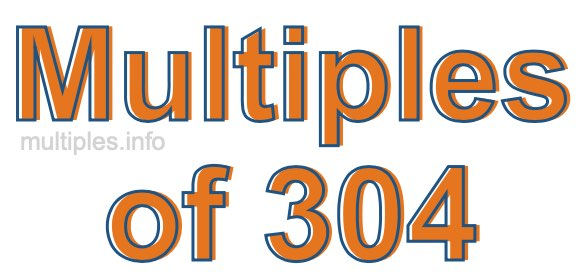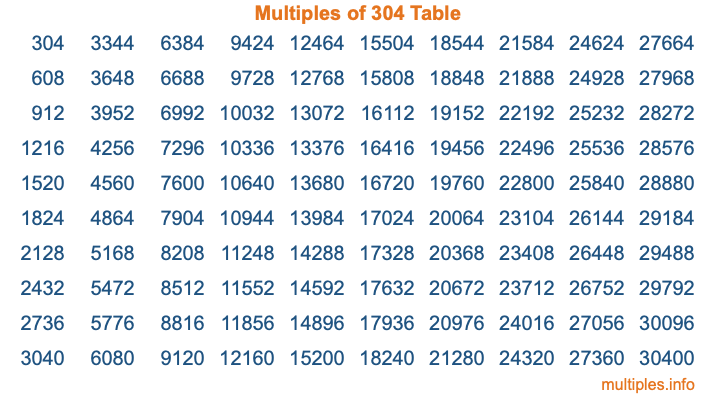Multiples of 304Welcome to the Multiples of 304 page. Here we will first teach you everything you will ever need to know about the multiples of 304, and then give you a study guide summary of everything we taught you to make sure you remember it all. Use this page to look up facts and learn information about the multiples of 304. This page will make you a multiples of three hundred four expert!

Definition of Multiples of 304
Multiples of 304 are all the numbers that when divided by 304 equal an integer. Each of the multiples of 304 are called a multiple. A multiple of 304 is created by multiplying 304 by an integer.

Therefore, to create a list of multiples of 304, you start with 1 multiplied by 304, then 2 multiplied by 304, then 3 multiplied by 304, and so on for as long as you want. Thus, the list of the first five multiples of 304 is 304, 608, 912, 1216, and 1520. To see a larger list of multiples of 304, see the printable image of Multiples of 304 further down on this page. We also have a category where you can choose any nth multiple of 304.

Multiples of 304 Checker
The Multiples of 304 Checker below checks to see if any number of your choice is a multiple of 304. In other words, it checks to see if there is any number (integer) that when multiplied by 304 will equal your number. To do that, we divide your number by 304. If the the quotient is an integer, then your number is a multiple of 304.

Is  a multiple of 304?

Least Common Multiple of 304 and ...
A Least Common Multiple (LCM) is the lowest multiple that two or more numbers have in common. This is also called the smallest common multiple or lowest common multiple and is useful to know when you are adding our subtracting fractions. Enter one or more numbers below (304 is already entered) to find the LCM.

Check out our LCM Calculator if you need more details about the Least Common Multiple or if you need the LCM for different numbers for adding and subtraction fractions.

nth Multiple of 304
As we stated above, 304 is the first multiple of 304, 608 is the second multiple of 304, 912 is the third multiple of 304, and so on. Enter a number below to find the nth multiple of 304.

th multiple of 304

Multiples of 304 vs Factors of 304
304 is a multiple of 304 and a factor of 304, but that is where the similarities end. All postive multiples of 304 are 304 or greater than 304. All positive factors of 304 are 304 or less than 304.

Below is the beginning list of multiples of 304 and the factors of 304 so you can compare:

Multiples of 304: 304, 608, 912, 1216, 1520, etc.

Factors of 304: 1, 2, 4, 8, 16, 19, 38, 76, 152, 304

As you can see, the multiples of 304 are all the numbers that you can divide by 304 to get a whole number. The factors of 304, on the other hand, are all the whole numbers that you can multiply by another whole number to get 304.

It's also interesting to note that if a number (x) is a factor of 304, then 304 will also be a multiple of that number (x).

Multiples of 304 vs Divisors of 304
The divisors of 304 are all the integers that 304 can be divided by evenly. Below is a list of the divisors of 304.

Divisors of 304: 1, 2, 4, 8, 16, 19, 38, 76, 152, 304

The interesting thing to note here is that if you take any multiple of 304 and divide it by a divisor of 304, you will see that the quotient is an integer.

Multiples of 304 Table
Below is an image of the first 100 multiples of 304 in a table. The table is in chronological order, column by column. The first column has the first ten multiples of 304, the second column has the next ten multiples of 304, and so on.The Multiples of 304 Table is also referred to as the 304 Times Table or Times Table of 304. You are welcome to print out our table for your studies.

Negative Multiples of 304
Although not often discussed or needed in math, it is worth mentioning that you can make a list of negative multiples of 304 by multiplying 304 by -1, then by -2, then by -3, and so on, to get the following list of negative multiples of 304:

-304, -608, -912, -1216, -1520, etc.

Multiples of 304 Summary
Below is a summary of important Multiples of 304 facts that we have discussed on this page. To retain the knowledge on this page, we recommend that you read through the summary and explain to yourself or a study partner why they hold true.

There are an infinite number of multiples of 304.

A multiple of 304 divided by 304 will equal a whole number.

304 divided by a factor of 304 equals a divisor of 304.

The nth multiple of 304 is n times 304.

The largest factor of 304 is equal to the first positive multiple of 304.

304 is a multiple of every factor of 304.

304 is a multiple of 304.

A multiple of 304 divided by a divisor of 304 equals an integer.

304 divided by a divisor of 304 equals a factor of 304.

Any integer times 304 will equal a multiple of 304.

Multiples of a Number
Here you can get the multiples of another number, all with the same attention to detail as we did for multiples of 304 on this page.

Multiples of
Multiples of 305
Did you find our page about multiples of three hundred four educational? Do you want more knowledge? Check out the multiples of the next number on our list!